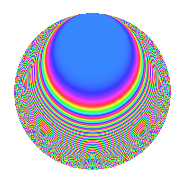# Properties

 Label 27.3.fLevel $27$ Weight $3$ Character orbit 27.f Rep. character $\chi_{27}(2,\cdot)$ Character field $\Q(\zeta_{18})$ Dimension $30$ Newform subspaces $1$ Sturm bound $9$ Trace bound $0$

# Related objects

## Defining parameters

 Level: $$N$$ $$=$$ $$27 = 3^{3}$$ Weight: $$k$$ $$=$$ $$3$$ Character orbit: $$[\chi]$$ $$=$$ 27.f (of order $$18$$ and degree $$6$$) Character conductor: $$\operatorname{cond}(\chi)$$ $$=$$ $$27$$ Character field: $$\Q(\zeta_{18})$$ Newform subspaces: $$1$$ Sturm bound: $$9$$ Trace bound: $$0$$

## Dimensions

The following table gives the dimensions of various subspaces of $$M_{3}(27, [\chi])$$.

Total New Old
Modular forms 42 42 0
Cusp forms 30 30 0
Eisenstein series 12 12 0

## Trace form

 $$30q - 6q^{2} - 6q^{3} - 6q^{4} - 15q^{5} - 18q^{6} - 6q^{7} - 9q^{8} + O(q^{10})$$ $$30q - 6q^{2} - 6q^{3} - 6q^{4} - 15q^{5} - 18q^{6} - 6q^{7} - 9q^{8} - 3q^{10} - 6q^{11} - 15q^{12} - 6q^{13} - 15q^{14} - 9q^{15} - 18q^{16} - 9q^{17} + 63q^{18} - 3q^{19} + 213q^{20} + 132q^{21} - 42q^{22} + 120q^{23} + 144q^{24} - 15q^{25} - 90q^{27} - 12q^{28} - 168q^{29} - 243q^{30} + 39q^{31} - 360q^{32} - 207q^{33} + 54q^{34} - 252q^{35} - 360q^{36} - 3q^{37} - 84q^{38} + 15q^{39} - 33q^{40} + 228q^{41} + 486q^{42} - 96q^{43} + 639q^{44} + 477q^{45} - 3q^{46} + 399q^{47} + 453q^{48} - 78q^{49} + 303q^{50} + 36q^{51} - 9q^{52} - 54q^{54} - 12q^{55} - 393q^{56} - 192q^{57} + 129q^{58} - 474q^{59} - 846q^{60} + 138q^{61} - 900q^{62} - 585q^{63} - 51q^{64} - 411q^{65} - 423q^{66} + 354q^{67} + 99q^{68} + 99q^{69} + 489q^{70} + 315q^{71} + 720q^{72} - 66q^{73} + 321q^{74} + 255q^{75} + 258q^{76} + 201q^{77} + 180q^{78} + 30q^{79} + 36q^{81} - 12q^{82} - 33q^{83} - 588q^{84} - 261q^{85} - 258q^{86} - 279q^{87} - 642q^{88} + 72q^{89} + 288q^{90} + 96q^{91} - 3q^{92} + 591q^{93} - 861q^{94} + 681q^{95} + 270q^{96} - 582q^{97} + 882q^{98} + 513q^{99} + O(q^{100})$$

## Decomposition of $$S_{3}^{\mathrm{new}}(27, [\chi])$$ into newform subspaces

Label Dim. $$A$$ Field CM Traces $q$-expansion
$$a_2$$ $$a_3$$ $$a_5$$ $$a_7$$
27.3.f.a $$30$$ $$0.736$$ None $$-6$$ $$-6$$ $$-15$$ $$-6$$

## Hecke characteristic polynomials

There are no characteristic polynomials of Hecke operators in the database